Search

The Pythagorean Theorem (page 2 of 2)

• Given the points (2, 3), (2, 1), and (5, 2), determine if they are the vertices of a right triangle.
• "Vertices" is the fancy word for "corners", so this is asking me to figure out if the three points are the three corners of a right triangle. From a quick graph, I can see that the points are the corners of some sort of triangle: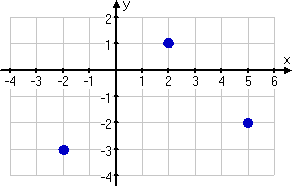I can use the Distance Formula to find the lengths of the each of the sides of the triangle, these lengths being the distances between the pairs of points. Then I can plus these distances into the Theorem to see if this triangle is a right triangle.

I think the points (2, 3) and (5, 2) mark off the hypotenuse, assuming this triangle turns out to be right, so I'll test the distances that way first.

(2, 3) and (2, 1):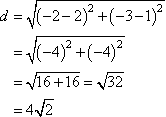(2, 1) and (5, 2):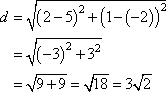(2, 3) and (5, 2):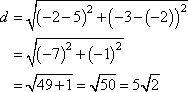checking the Theorem (using the squares of the distances):

32 + 18 = 50

Since the squares of the smaller two distances equal the square of the largest distance, then these points are the vertices of a right triangle.

• Zedekiah is building a gate. It is to be five feet tall and eight feet wide. If the gate is "square" (that is, if the sides meet at the corners to form right angles), what will be the length of the diagonal bracing wire? Round to the nearest quarter-inch.
• The width (going horizontally), the height (going vertically), and the wire (going diagonally across) for the gate form a right triangle. When the gate is square, the diagonal will obey the Pythagorean Theorem. Copyright © Elizabeth Stapel 2010-2011 All Rights Reserved

52 + 82 = c2
25 + 64 = 89 = c2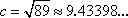So the bracing wire will be nine feet long, plus another 0.43389 feet or so. There are twelve inches in one foot, so:

0.43389 × 12 = 5.20776

There will be another about 5.2 inches; 0.2 is closer to 0.25 than to 0.0, so:

The length of the wire will be 9 feet, 5 1/4 inches.

 Zedekiah needs to find the width of the pond on a plot of land he's selling. He has made measurements to point R from points P and Q on either side of the pond, and is certain that the angle at P is right, as displayed: Assuming his measurements are correct, what is the width of the pond? I have two sides of what is to be assumed to be a right triangle, so I can apply the Pythagorean Theorem to find the length of the third side. Since the angle at P is the (assumed) right angle, then QR is the hypotenuse, and: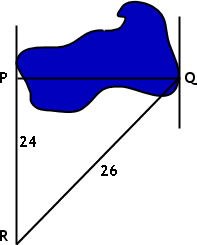262 = 242 + |PQ|2
676 = 576 + |PQ|2

100 = |PQ|2

10 = |PQ|

The pond is ten meters across.

Note that, for the above answer to be correct, the displayed picture can not possibly be "to scale". You should assume, as a matter of course, that the pictures for geometry and trigonometry exercises are not "to scale". Do not make the mistake of trying to guess the answers from the pictures!